Matrices, vectors, addition, scalar multiplication, matrix vector multiplication, matrix matrix multiplication, properties of matrix multiplication, inverse matrix and transposing matrices.

## 1. Matrices and Vectors

I would like to give full credits to the respective authors as these are my personal python notebooks taken from deep learning courses from Andrew Ng, Data School and Udemy :) This is a simple python notebook hosted generously through Github Pages that is on my main personal notes repository on https://github.com/ritchieng/ritchieng.github.io. They are meant for my personal review but I have open-source my repository of personal notes as a lot of people found it useful.

### 1a. Matrices

• Rectangular array of numbers
• 2D array
• Number of Rows x Number of Columns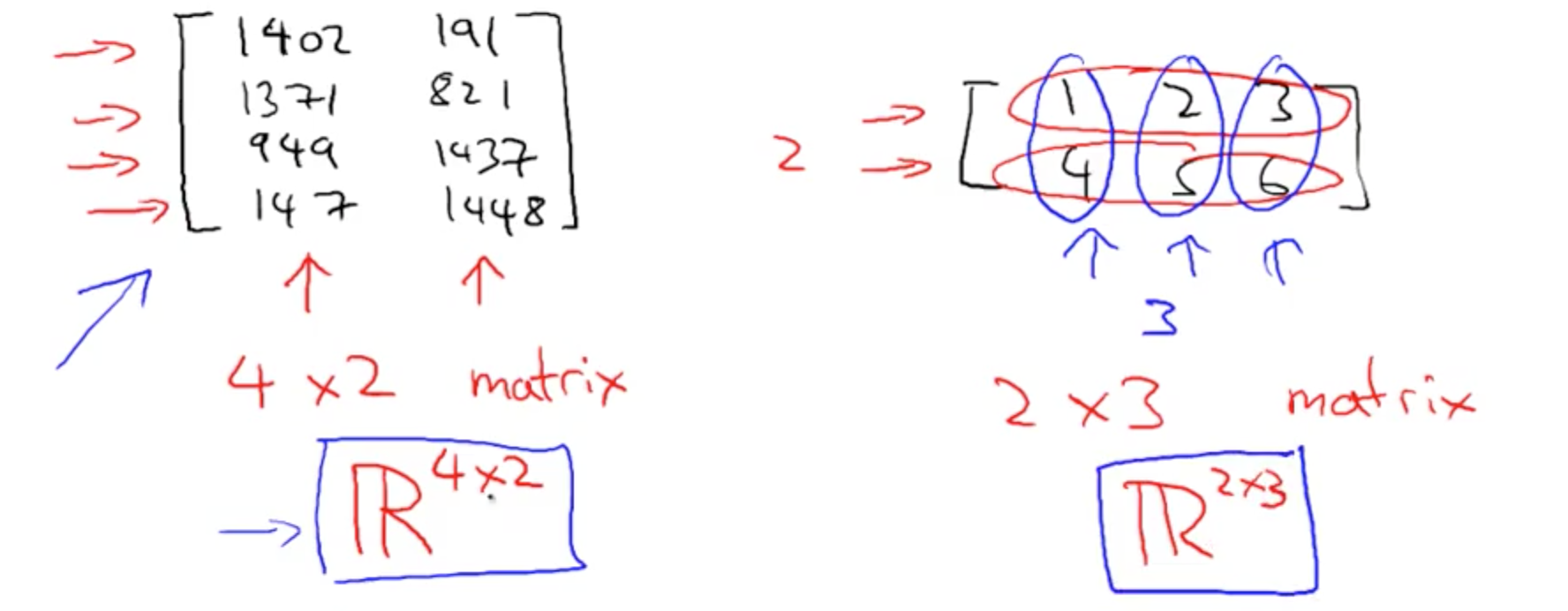### 1b. Vector

• n x 1 matrix
• y(i): i-th element
• 1-indexed (start from 1-th)
• Normally this
• 0-indexed (start from 0-th)
• Used in Machine Learning

## 2. Addition and Scalar Multiplication

• You can only add matrices with the same dimensions (r x c)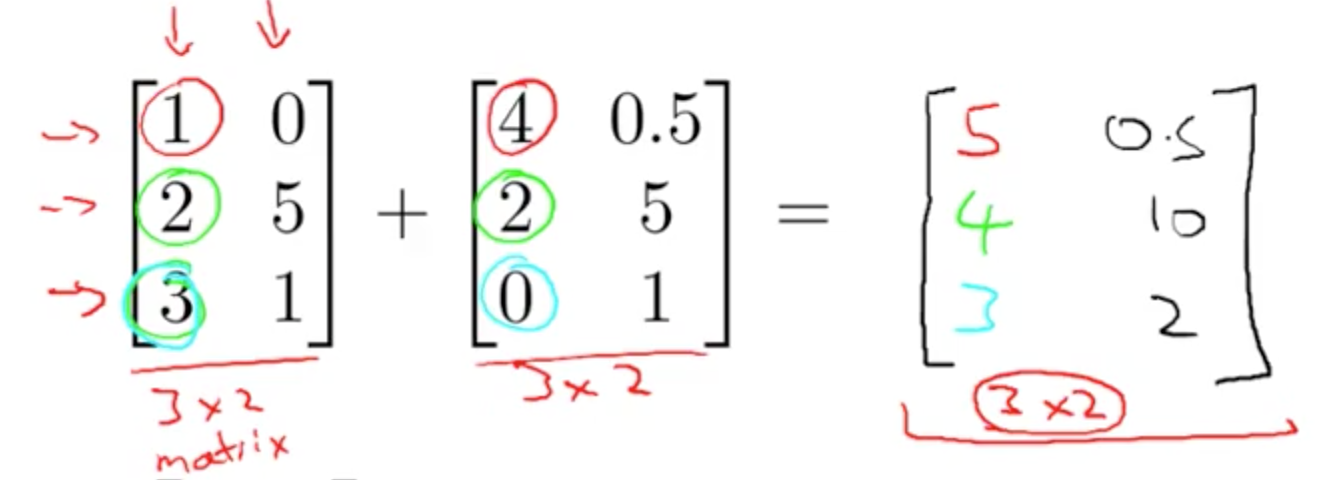### 2b. Scalar (Number) Multiplication

• Example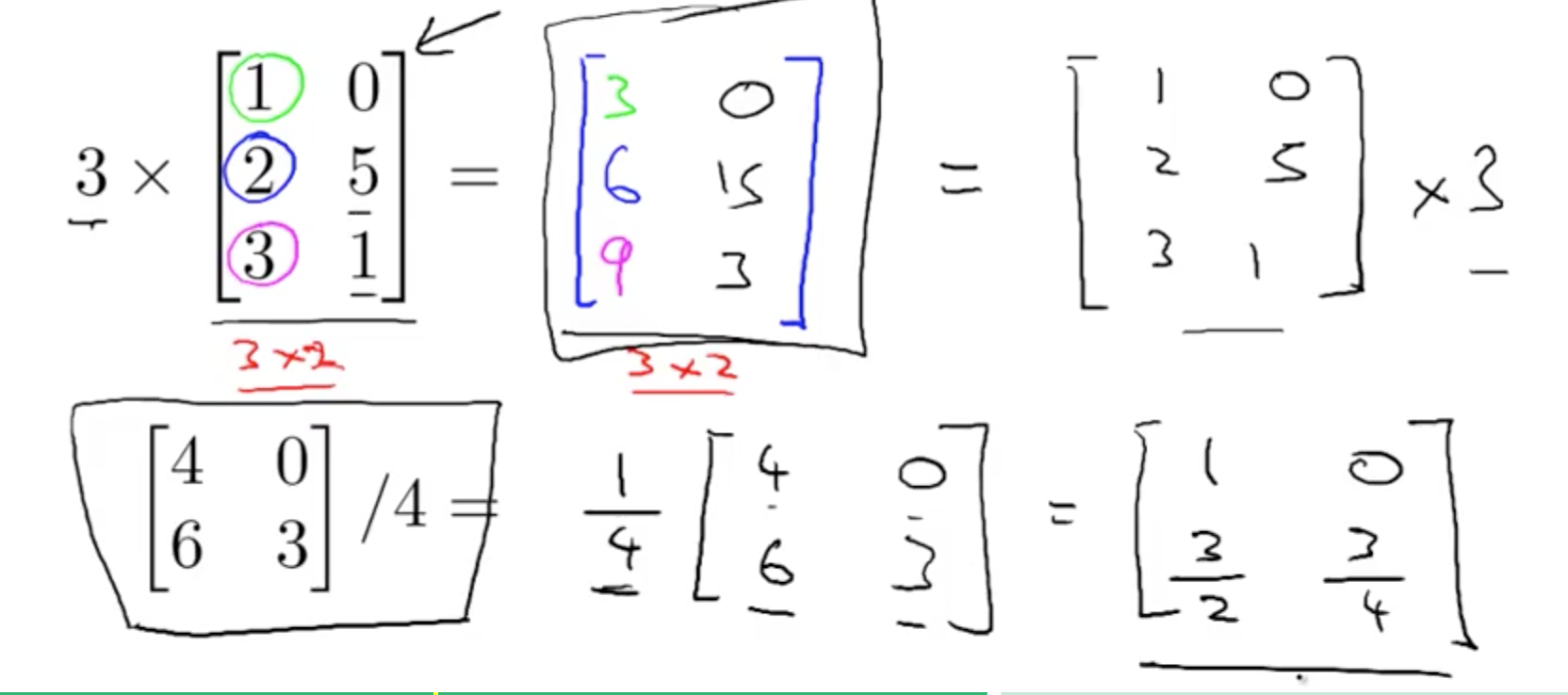## 3. Matrix Vector Multiplication

• Example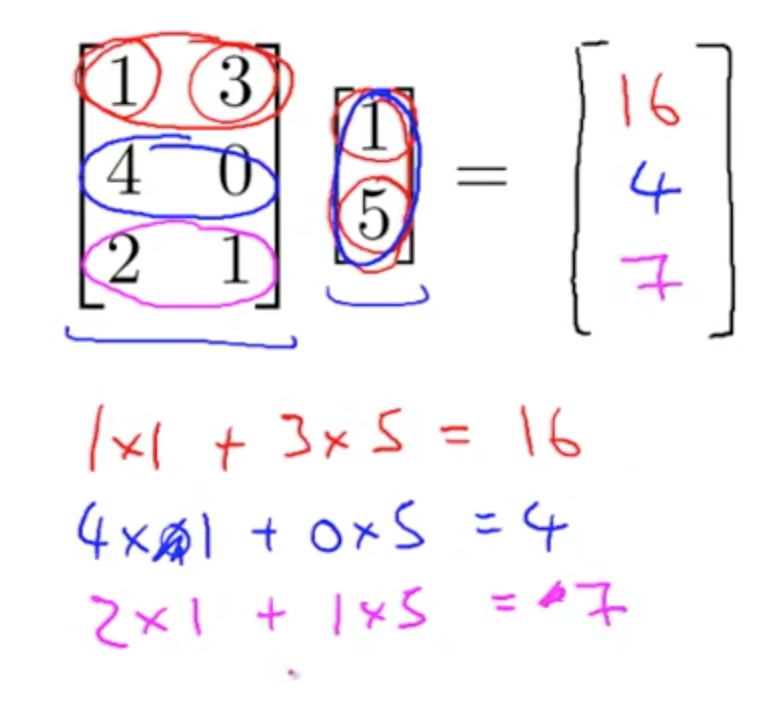• Theory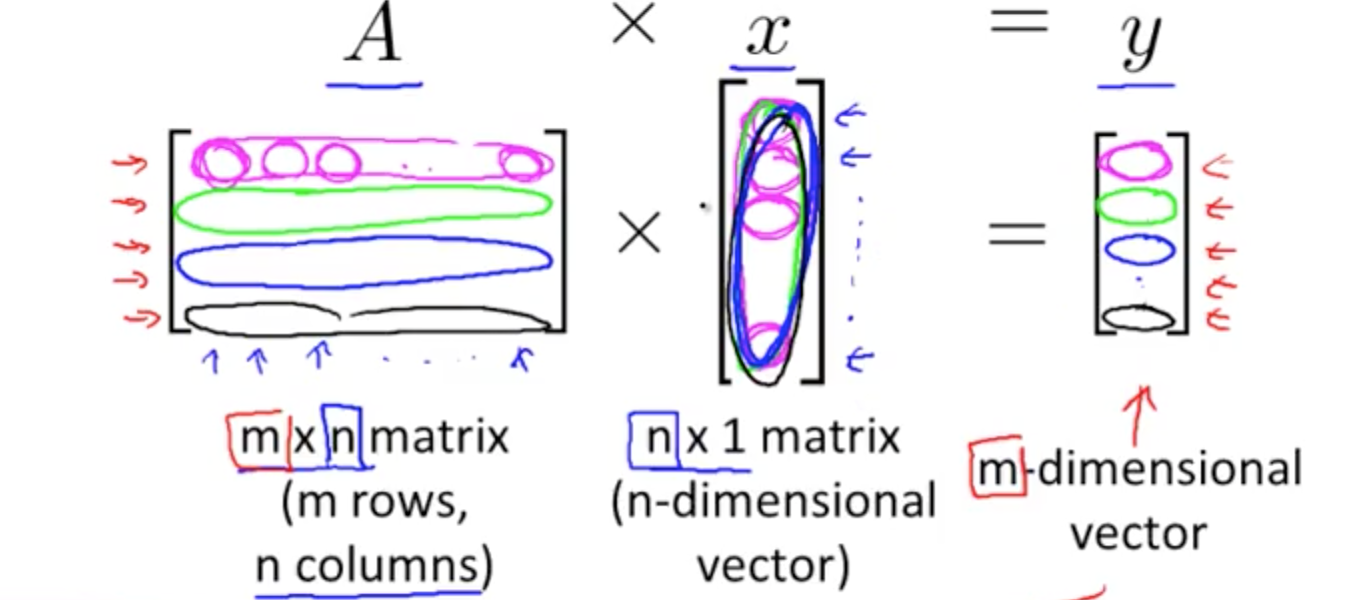• Application to hypothesis by converting given data to matrix
• prediction = data_matrix x parameters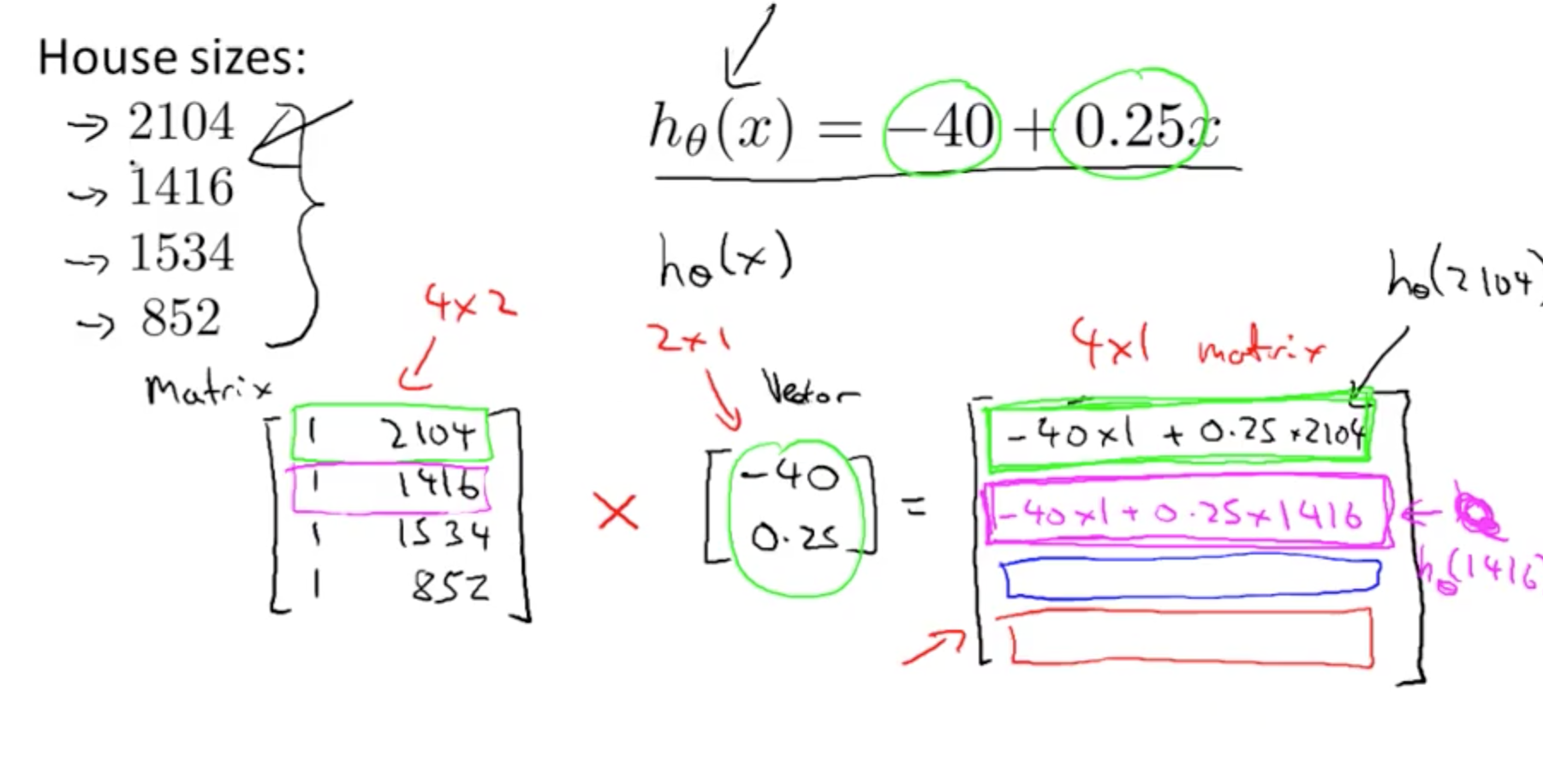## 4. Matrix Matrix Multiplication

• Example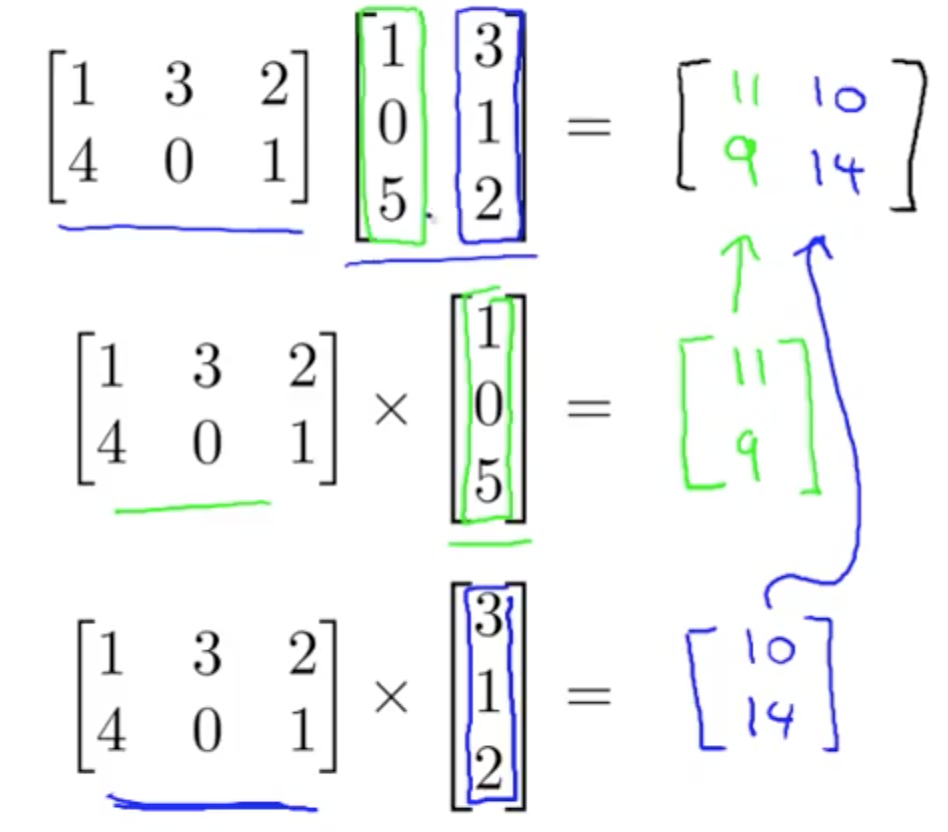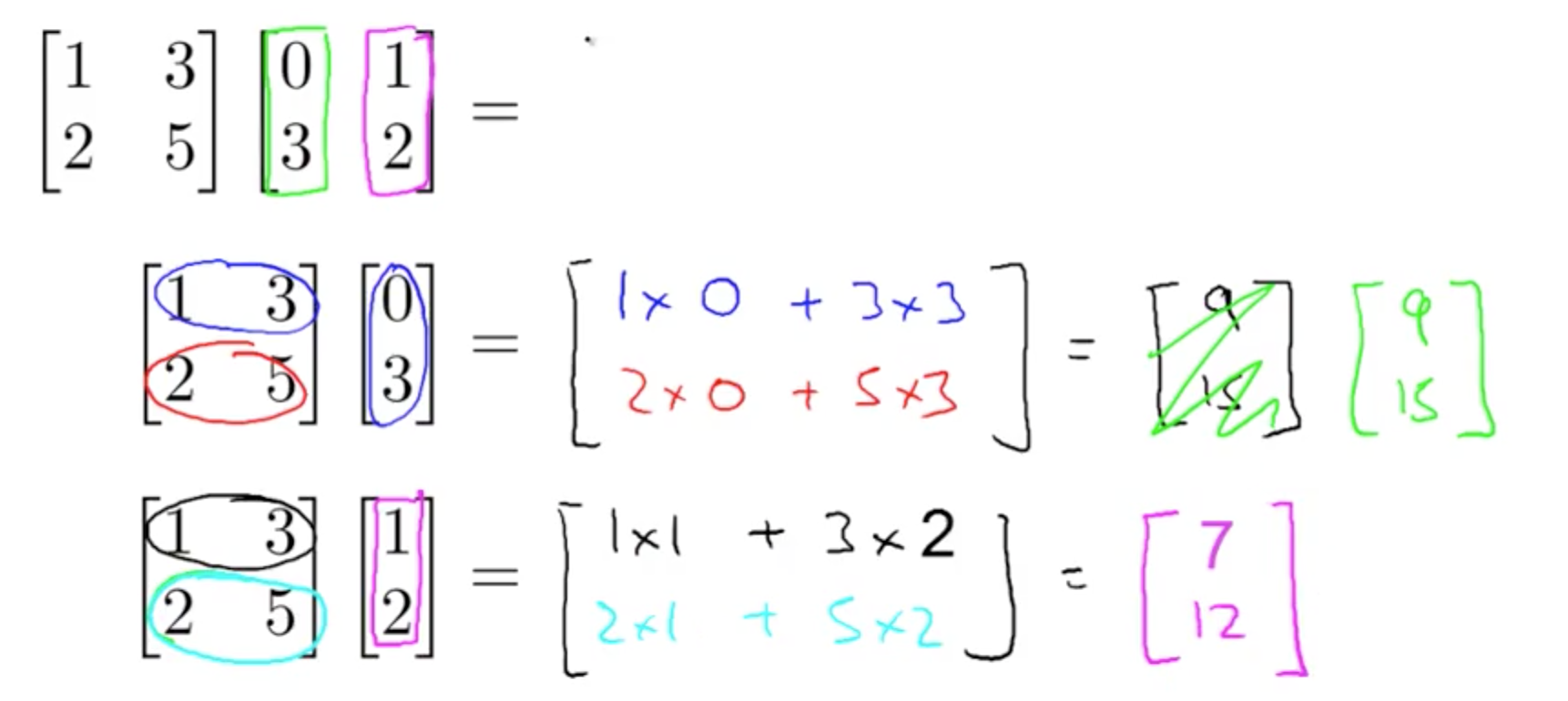• Theory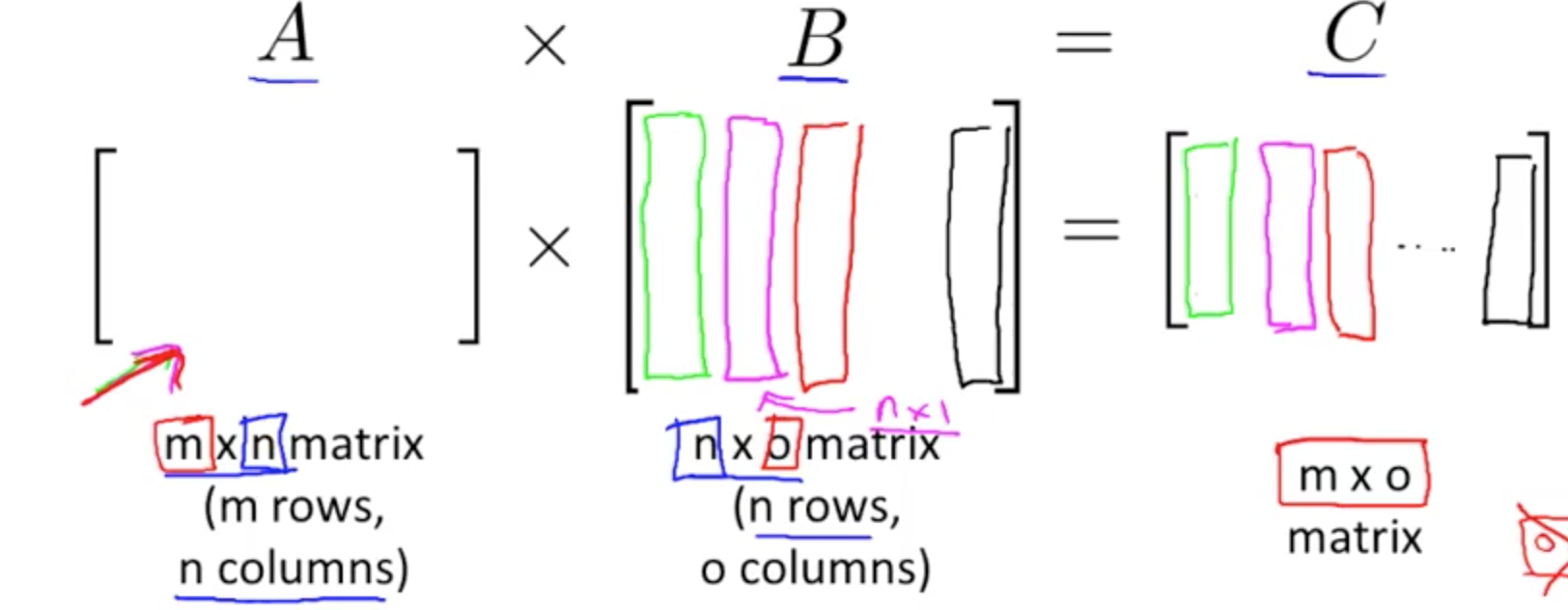• Application to hypothesis by converting given data to matrix
• There are linear algebra libraries to do these calculations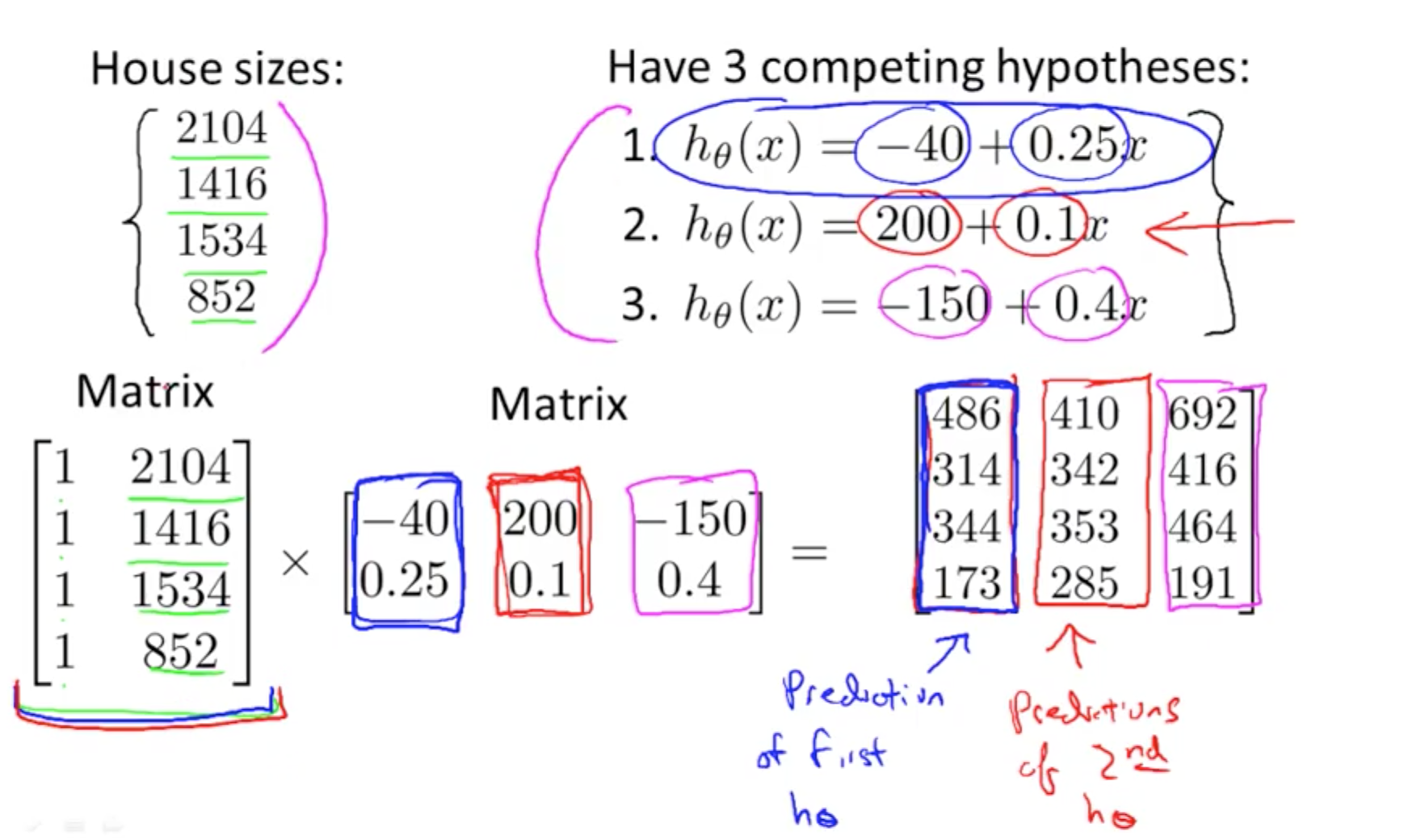## 5. Properties of Matrix Multiplication

• Not commutative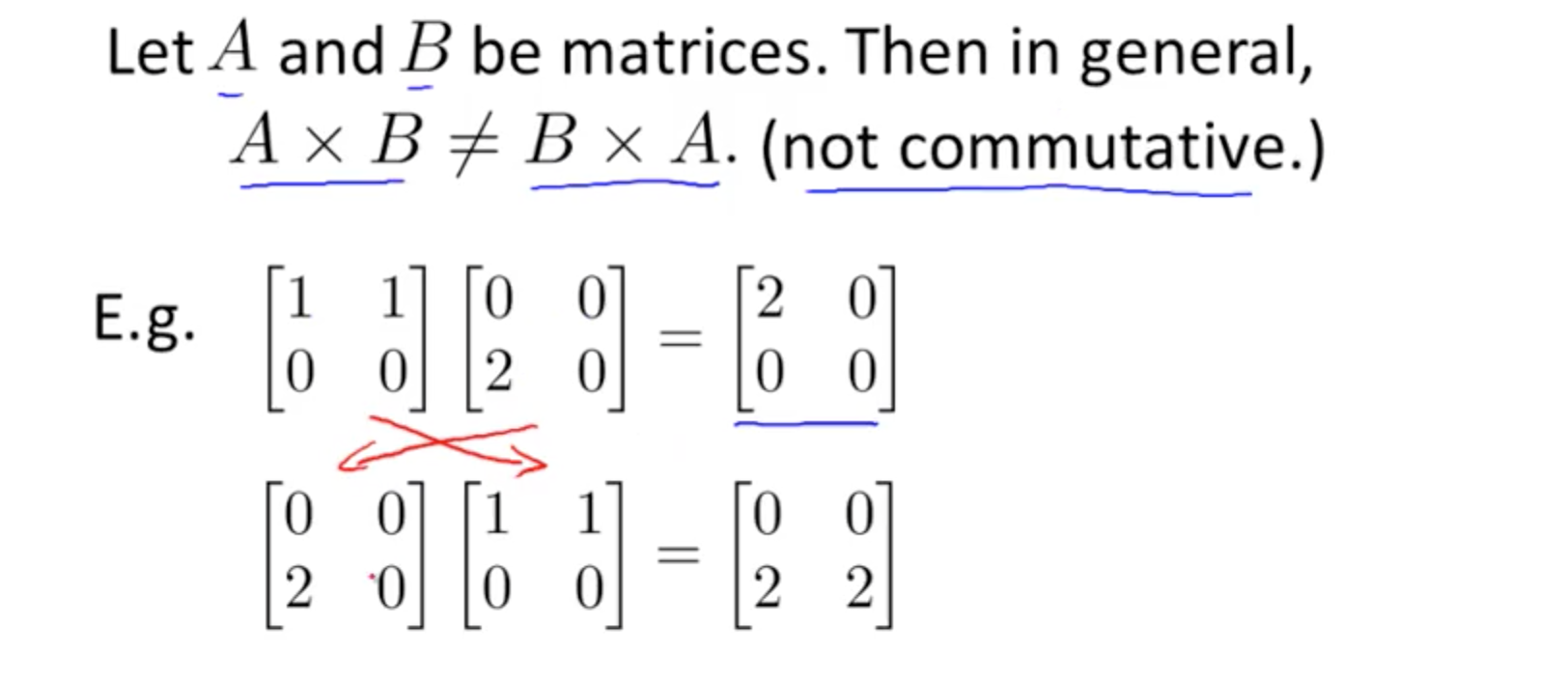• Associative
• A x B x C = (A x B) x C = A x (B x C)
• Identity Matrix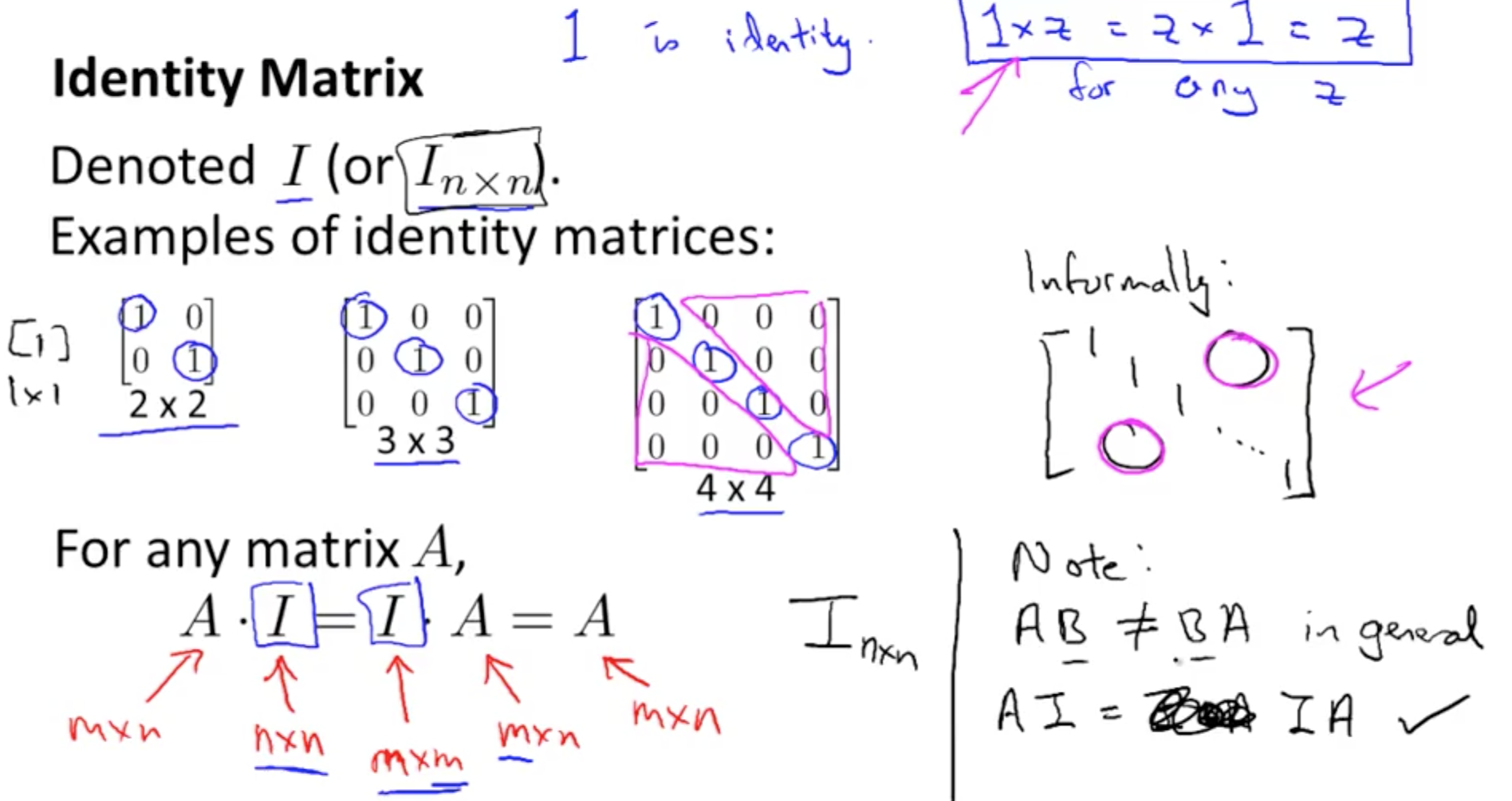## 6. Inverse and Transpose

### 6a. Inverse

• A * A_inverse = Identity Matrix
• A_inverse = pinv(A)
• You can use octave code pinv(A)
• Matrices without inverse –> singular or degenerate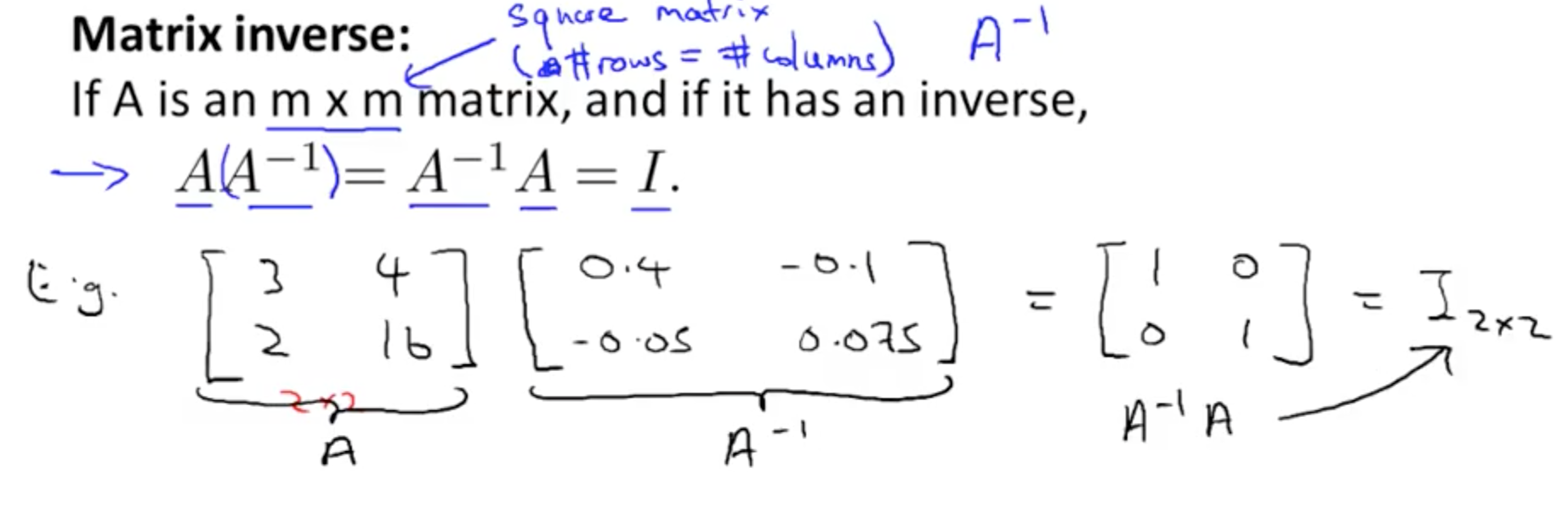### 6b. Transpose

• Example and theory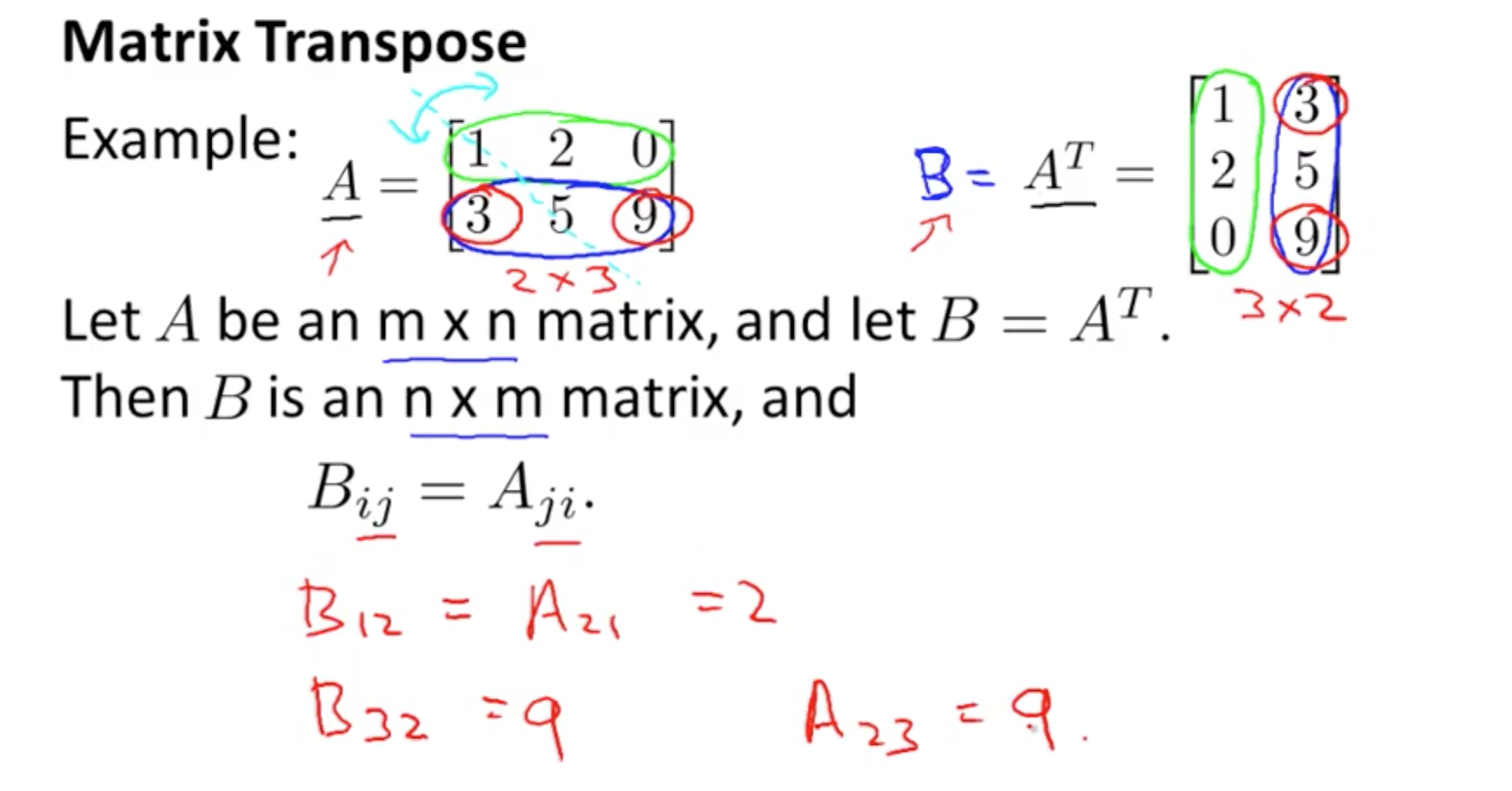Tags: